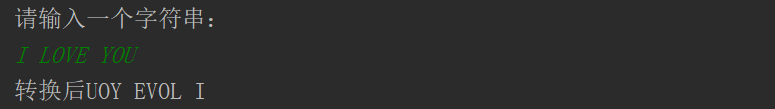• 思想：用栈进行字符串逆序。首先字母从输入的字符串中开一个一个的提取出来，并压入栈中，接着它们依次弹出栈，并显示出来，因为栈后进先出的特性，字符串的顺序就颠倒过来了。 StackX类 public class StackX { ...
要求：提示输入一个字符串，回车后屏幕显示字符串顺序倒置后的结果。 思想：用栈进行字符串逆序。首先字母从输入的字符串中开一个一个的提取出来，并压入栈中，接着它们依次弹出栈，并显示出来，因为栈后进先出的特性，字符串的顺序就颠倒过来了。
StackX类
public class StackX {
private int max_size;           //栈最大容量
private char[] stackArray;
private int top;                //栈顶下标
public StackX(int m){           //构造方法
max_size=m;
stackArray=new char[max_size];
top=-1;
}
public void push(char p1){     //进栈
stackArray[++top]=p1;
}
public char pop(){
return stackArray[top--]; //出栈
}
public char peek(){          //查看栈顶元素
return stackArray[top];
}
public boolean isEmpty(){   //判断栈是否为空
return (top==-1);
}
public boolean isFull(){   //判断是否已满
return (top==max_size-1);
}
}

Reverser类
public class Reverser {
private String input;
private String output;
public Reverser(String s){
input=s;
}
public String doRec(){                      //字符串倒序方法
int max_size=input.length();
StackX s=new StackX(max_size);          //创建大小为输入字符串长度的栈
for (int i=0;i<input.length();i++){
char c1=input.charAt(i);
s.push(c1);                         //循环入栈
}
output="";
while(!s.isEmpty()){
char c2=s.pop();                    //出栈
output=output+c2;                   //字符串拼接，可直接用"+"
}
return output;
}
}

测试类RecerserApp
public class RecerserApp {
public static void main(String args[]){
String input,output;
while (true) {
System.out.println("请输入一个字符串：");
System.out.flush();
//flush();是流式输入输出常用的一个方法，表示强制请求清空缓冲区，让i/o系统立马完成它应该完成的输入、输出动作。
input=getString();               //接收从控制台输入的字符串
if(input.equals("")){
break;
}
Reverser theReverser=new Reverser(input);
output=theReverser.doRec();
System.out.println("转换后"+output);
}
}
/*
System.in 接受从控制台输入的字节。
这个对象是字节流通向字符流的桥梁：它使用指定的 charset 读取字节并将其解码为字符。
构造一个字符流的缓存，里面存放在控制台输入的字节转换后成的字符。

依次类推 一种低级字节流管道的管道 我们可以在其外面嵌套一个更高级的数据流管道 从而更加方便的读写数据
*/
public static  String getString(){
String s= null;
try {
} catch (IOException e) {
e.printStackTrace();
}
return s;
}
}

运行结果：展开全文倒序 字符串倒序
• 这篇文章主要介绍了python实现对指定输入字符串逆序输出的6种方法，具有一定的参考价值，感兴趣的小伙伴们可以参考一下对于一个给定的字符串，逆序输出，这个任务对于python来说是一种很简单的操作，毕竟强大的...
这篇文章主要介绍了python实现对指定输入的字符串逆序输出的6种方法，具有一定的参考价值，感兴趣的小伙伴们可以参考一下
对于一个给定的字符串，逆序输出，这个任务对于python来说是一种很简单的操作，毕竟强大的列表和字符串处理的一些列函数足以应付这些问题 了，今天总结了一下python中对于字符串的逆序输出的几种常用的方法，一共总结出来了六种方法，个人认为比较全面了，如有其他方法欢迎前来补充
方法一：直接使用字符串切片功能逆转字符串
方法二：将字符串转换为列表使用reverse函数
方法三：新建一个列表，从后往前添加元素
方法四：借助于collections模块现成的方法extendleft
方法五：递归实现
方法六：借助基本的Swap操作，以中间为基准交换对称位置的字符
下面是具体的实现：
#!usr/bin/env python
#encoding:utf-8
'''''''
__Author__:沂水寒城
功能：输入一个字符串，倒叙输出
'''
import collections
def func1(one_str):
'''''
直接使用字符串切片功能逆转字符串
'''
return one_str[::-1]
def func2(one_str):
'''''
将字符串转换为列表使用reverse函数
'''
one_str_list = list(one_str)
one_str_list.reverse()
return ''.join(one_str_list)
def func3(one_str):
'''''
新建一个列表，从后往前添加元素
'''
one_list=[]
for i in range(len(one_str)-1,-1,-1):
one_list.append(one_str[i])
return ''.join(one_list)
def func4(one_str):
'''''
借助于collections模块现成的方法extendleft
'''
deque1=collections.deque(one_str)
deque2=collections.deque()
for one_char in deque1:
deque2.extendleft(one_char)
return ''.join(deque2)
def func5(one_str):
'''''
递归实现
'''
if len(one_str)<=1:
return one_str
return one_str[-1]+func5(one_str[:-1])
def func6(one_str):
'''''
借助基本的Swap操作，以中间为基准交换对称位置的字符
'''
one_str_list=list(one_str)
if len(one_str_list)==0 or len(one_str_list)==1:
return one_str_list
i=0
length=len(one_str_list)
while i < length/2:
one_str_list[i], one_str_list[length-i-1]=one_str_list[length-i-1], one_str_list[i]
i+=1
return ''.join(one_str_list)
def main_func(str_list):
'''''
主调用函数
'''
for one_str in str_list:
one_list=[]
one=[]
one_list.append(func1(one_str))
one_list.append(func2(one_str))
one_list.append(func3(one_str))
one_list.append(func4(one_str))
one_list.append(func5(one_str))
one_list.append(func6(one_str))
print '字符串{0}逆序为：'.format(one_str)
print one_list
if __name__ == '__main__':
str_list=['123456','abcdefg','zyxvuw','Together_CZ']
main_func(str_list)
结果如下：
字符串123456逆序为：
['654321', '654321', '654321', '654321', '654321', '654321']
字符串abcdefg逆序为：
['gfedcba', 'gfedcba', 'gfedcba', 'gfedcba', 'gfedcba', 'gfedcba']
字符串zyxvuw逆序为：
['wuvxyz', 'wuvxyz', 'wuvxyz', 'wuvxyz', 'wuvxyz', 'wuvxyz']
字符串Together_CZ逆序为：
['ZC_rehtegoT', 'ZC_rehtegoT', 'ZC_rehtegoT', 'ZC_rehtegoT', 'ZC_rehtegoT', 'ZC_rehtegoT']
相关推荐：
以上就是python实现对指定输入的字符串逆序输出的方法的详细内容，更多请关注php中文网其它相关文章！本文原创发布php中文网，转载请注明出处，感谢您的尊重！
展开全文• 对于一个给定的字符串逆序输出，这个任务对于python来说是一种很简单的操作，毕竟强大的列表和字符串处理的一些列函数足以应付这些问题 了，今天总结了一下python中对于字符串逆序输出的几种常用的方法，一共...

对于一个给定的字符串，逆序输出，这个任务对于python来说是一种很简单的操作，毕竟强大的列表和字符串处理的一些列函数足以应付这些问题 了，今天总结了一下python中对于字符串的逆序输出的几种常用的方法，一共总结出来了六种方法，个人认为比较全面了，如有其他方法欢迎前来补充
方法一：
直接使用字符串切片功能逆转字符串
方法二：
将字符串转换为列表使用reverse函数
方法三：
新建一个列表，从后往前添加元素
方法四：
借助于collections模块现成的方法extendleft
方法五：
递归实现
方法六：
借助基本的Swap操作，以中间为基准交换对称位置的字符
下面是具体的实现：
#!usr/bin/env python #encoding:utf-8  ''''' __Author__:沂水寒城 功能：输入一个字符串，倒叙输出'''  import collections   def func1(one_str): ''' 直接使用字符串切片功能逆转字符串 ''' return one_str[::-1]  def func2(one_str): ''' 将字符串转换为列表使用reverse函数 ''' one_str_list = list(one_str) one_str_list.reverse() return ''.join(one_str_list)  def func3(one_str): ''' 新建一个列表，从后往前添加元素 ''' one_list=[] for i in range(len(one_str)-1,-1,-1): one_list.append(one_str[i]) return ''.join(one_list)  def func4(one_str): ''' 借助于collections模块现成的方法extendleft ''' deque1=collections.deque(one_str) deque2=collections.deque() for one_char in deque1: deque2.extendleft(one_char) return ''.join(deque2)  def func5(one_str): ''' 递归实现 ''' if len(one_str)<=1: return one_str  return one_str[-1]+func5(one_str[:-1])  def func6(one_str): ''' 借助基本的Swap操作，以中间为基准交换对称位置的字符 ''' one_str_list=list(one_str) if len(one_str_list)==0 or len(one_str_list)==1: return one_str_list i=0 length=len(one_str_list) while i < length/2: one_str_list[i], one_str_list[length-i-1]=one_str_list[length-i-1], one_str_list[i] i+=1 return ''.join(one_str_list)  def main_func(str_list): ''' 主调用函数 ''' for one_str in str_list: one_list=[] one=[] one_list.append(func1(one_str)) one_list.append(func2(one_str)) one_list.append(func3(one_str)) one_list.append(func4(one_str)) one_list.append(func5(one_str)) one_list.append(func6(one_str)) print '字符串{0}逆序为：'.format(one_str) print one_list  if __name__ == '__main__': str_list=['123456','abcdefg','zyxvuw','Together_CZ'] main_func(str_list)
结果如下：
字符串123456逆序为：['654321', '654321', '654321', '654321', '654321', '654321']字符串abcdefg逆序为：['gfedcba', 'gfedcba', 'gfedcba', 'gfedcba', 'gfedcba', 'gfedcba']字符串zyxvuw逆序为：['wuvxyz', 'wuvxyz', 'wuvxyz', 'wuvxyz', 'wuvxyz', 'wuvxyz']字符串Together_CZ逆序为：['ZC_rehtegoT', 'ZC_rehtegoT', 'ZC_rehtegoT', 'ZC_rehtegoT', 'ZC_rehtegoT', 'ZC_rehtegoT']展开全文• 编写一个Python程序，提示用户输入一个字符串，程按照你的要分享编写的逆序显示字符串的Python3程序如下 s = input("请输入一个字符串...输入一个字符串+把其中的字符按逆序输出#include #include void main(void) {...
编写一个Python程序，提示用户输入一个字符串，程按照你的要分享编写的逆序显示字符串的Python3程序如下 s = input("请输入一个字符串:")print(s[::-1]) #s[::-1]相当于s[-1:-len(s)-1:-1],也就是从最后一个元素到第一个元素复制一遍。
输入一个字符串+把其中的字符按逆序输出
#include #include void main(void) { char s#include #include void main(void) { char s,ch; int len,i; gets(s); len=strlen(s); for(i=0;i小编爱你，是多么清楚多么坚固的信仰。小编爱你，是多么温暖多么勇敢的力量。
Python逆序输出怎么搞，急分享if __name__ == "__main__": string = input("请输入一个字符串：") string = string.split() string_re = string[::-1] for s in string_re: print(s,end='') print() print(string) for s in string_re: print(s,end=' ') print() 望喜欢。
编写一个Python程序，提示用户输入一个字符串，程下面是一个运行示例： Enter a string: Hello, World。 The reversed strEnter a string: Str='Hello,World。'The reversed string is: Str[::-1]不在乎你的人，你做的再好，再完美他也不在乎，因为无心，更因为无情。
如何用string方法解决“输入一个字符串，把其中的字详细方法和步骤： 1，定义和构造初始化：string 提供了很多构造函数，可以以多种方式来初始化string字符串 2，赋值，拼接字符串：string重载了 = + += 等多种运算符，让字符串组合拼接更简单 3，访问字符操作：string可以按数组方式。
7-10 字符串逆序 python计算机编程题
7-10 字符串逆序 （5 分） 输入一个字符串，对该字符串进行逆序，输出逆lin1=[]for i in range(1,11): lin1.append(i)lin1.reverse()print(lin1)往事是一道风景，总会在记忆的年华里低吟浅唱，那些曾经身手相牵的过往，那些洒落在岁月中的人生初识，即便隔着光阴的距离，却也会在心底，温暖一生，怀念一生。
输入一个字符串，把其中的字符按逆序输出，如果输#include #include using namespace std; int mainint main() { string str; int i,n; char temp; cout当你说出要小编坚强的时候，小编就明白了你最后的选择。
编写一程序：实现将现有字符串逆序输出。第一次哭泣是因为心疼渐渐成了回忆，第一次想你是因为孤单渐渐成了需要。
编写一程序：实现将现有字符串逆序输出。 比如：原有字符串abcde，输#include #include main(){ char s; int i=0; printf("输入一个字符串：\n?"); while((s[i]=getchar())。='\n') i++; while (i>=0){ putchar(s[i]); i; } printf("\n"); system("pause"); } 以上代码在dev c++中通过测试。
输入一个字符串，把其中的字符按逆序输出。如输入L欢迎来到四十五资源网，以下使用了string类以及反向枚举器实现逆序输出： #include #include using namespace std; void main() { string str; coutstr; // 获得反向的枚举器，反向遍历 string::reverse_iterator iter = str.rbegin(); cout所谓猪一样的闺密是买鞋子买到一双同一只脚的鞋子.
展开全文• 要求删除每个字符串中的字符ch(区分大小写)，得到新的字符串，然后将新的字符串按照字典逆序排序后输出(每个字符串的长度不超过30个字符，字符串总数不超过30)输入：3abcddcsxwcdezncvccvdc输出：sxwdeznvvdabddC++...
• 主要介绍了python实现对指定输入字符串逆序输出的6种方法，具有一定的参考价值，感兴趣的小伙伴们可以参考一下
• 要求删除每个字符串中的字符ch(区分大小写)，得到新的字符串，然后将新的字符串按照字典逆序排序后输出(每个字符串的长度不超过30个字符，字符串总数不超过30)输入：3 abcddc sxwcdez ncvccvd c输出：sxwdez nvvd ...
• 对于一个给定的字符串逆序输出，这个任务对于python来说是一种很简单的操作，毕竟强大的列表和字符串处理的一些列函数足以应付这些问题 了，今天总结了一下python中对于字符串逆序输出的几种常用的方法，一共...
•汇编
• 使用逆序来排列字符串并判断输入字符串是否为回文
• 项目要求是这样的，定义一个类，要求在实现迭代器功能的基础上，能够对用户输入字符串逆序输出操作。实现思路是这样的，自定义一个类并重载其 __init__() 初始化方法，实现为自身私有成员赋值。同时重载 __iter_...
• 输入字符串逆序输出 这里选择使用gets()，因为使用scanf()和%s的时候空格是作为分隔符，所以当输入“hello world！”这样的句子的时候world！会输入不进去（当然可以使用scanf和%c，这里选择gets()最简便） #...
• 【问题描述】将输入字符串逆序输出输入形式】要求输入一个字符串 【输出形式】逆序后输出 方法一： #include<stdio.h> #include<string.h> int main() { char a,b; int i,n; scanf("%s",...
• 举例：#includeint main(){int i, j, cnt;char ch, a, b;ch=getchar();for(i=0; ch!='\n'; i++){a[i]=ch;cnt++;ch=getchar();}for(i=0, j=cnt-1; ib[j]=a[i];for(i=0;...}运行：输出格式：在...
• 主要介绍了Python实现字符串逆序输出功能,结合具体实例形式分析了Python针对字符串的遍历、翻转、排序等相关操作技巧,需要的朋友可以参考下
• 利用c++实现string类字符串单词的逆序输出，并改变源字符串的大小写。#include#include#includeusing namespace std;string StringReverseWord(string &s){int len = s.length();if (len == 0)//空字符串return ...
• 从键盘上输入一组字符串，实现逆序输出。 解题思路 方法一：输出的时候从（n-1）的数据往前依次输出。 方法二：假设输入n个字符，字符串默认末尾补上' \0 '，将第0个字符和第（n-1）个字符交换，依次交换中间的...
• C++编程：输入一个字符串，把其中的字符按逆序输出。如输入LIGHT，输出THGIL.1. 用字符数组方法；2. 用string 方法以下是此题的【c++源代码】1. 用字符数组方法的【c++源代码】#include using namespace std;int ......